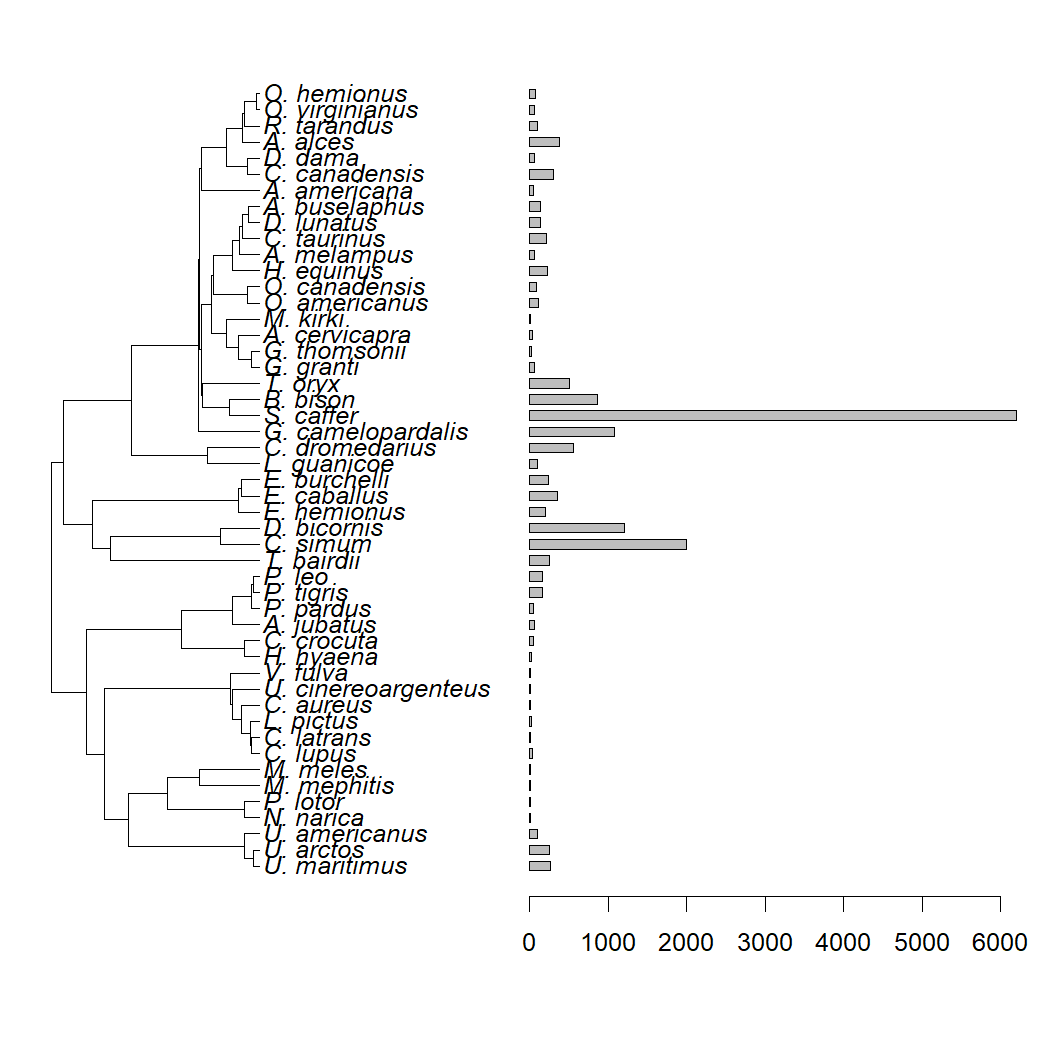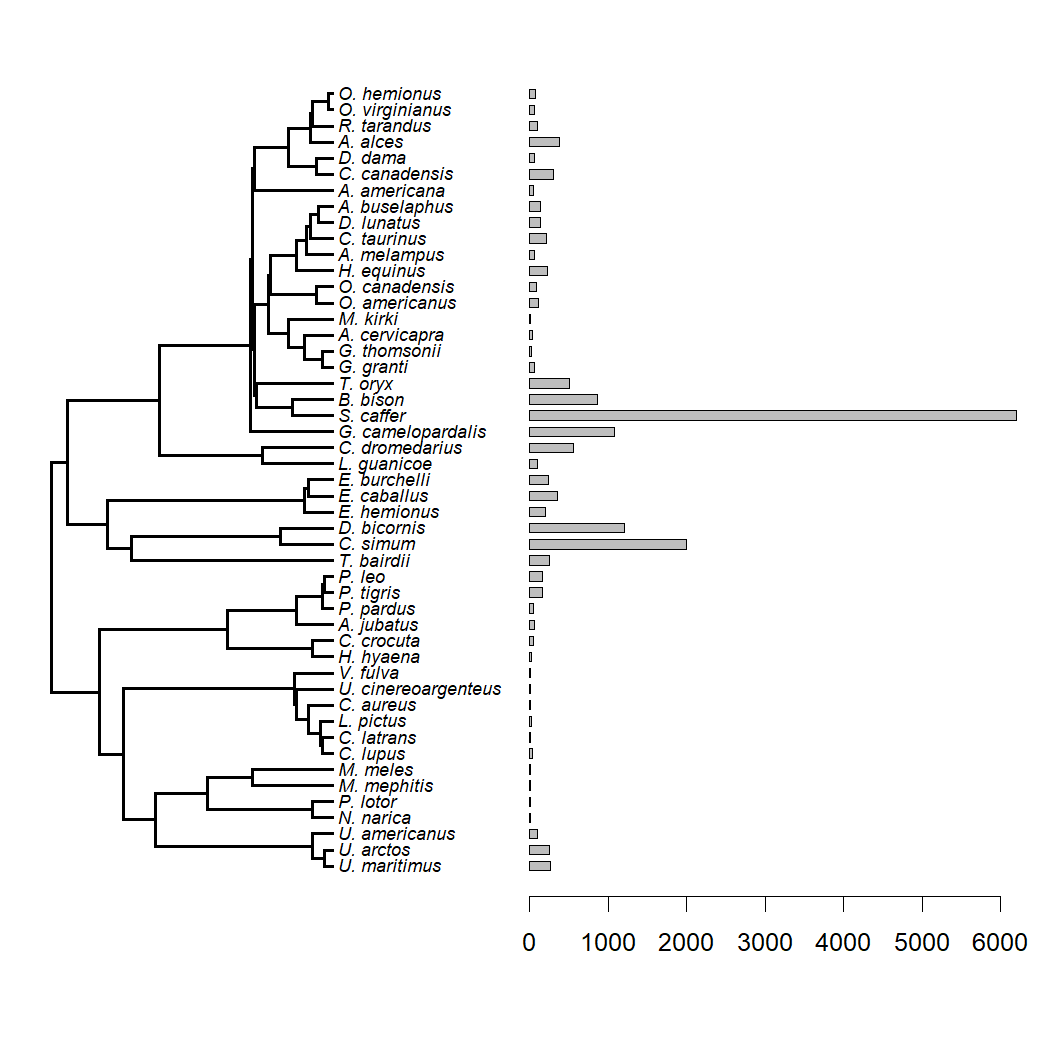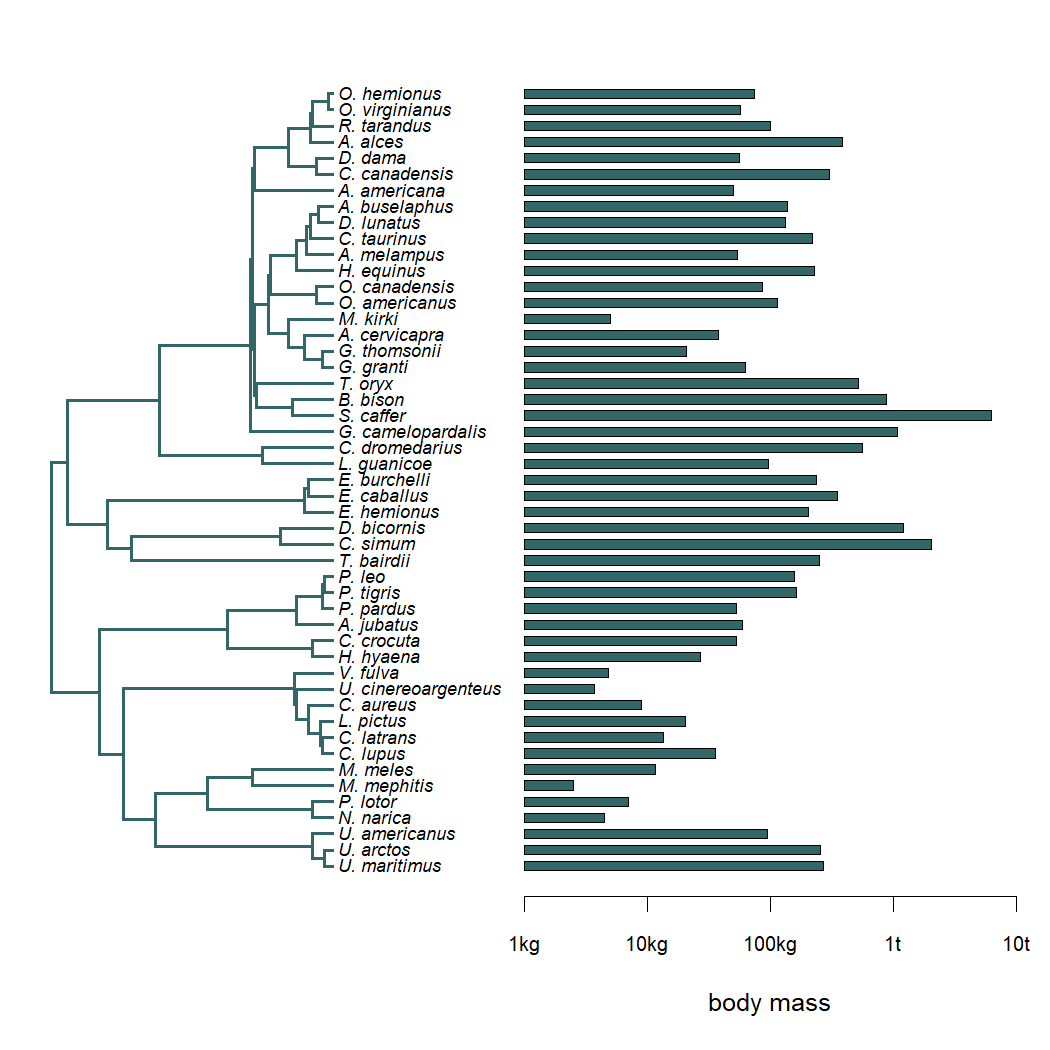## Wednesday, August 4, 2021

### Control subplot settings in `plotTree.barplot`

“I have been using mostly the `plotTree.barplot` function of phytools but one thing I find difficult is how to manipulate the arguments that modify the appearance of the tree graph. I cannot find documentation that is clearly described in one place on how to manipulate for example the legend size, location or the distances among the bars. Whatever I have found is through existing examples on the internet or your blog. Is there somewhere documentation on available arguments for customizing the appearance of graphs (e.g., legend size, position, bar thickness etc)?”

`plotTree.barplot` has quite a lot of flexibility to control the options for both the plotted tree & the barplot, although it is not fully flexible.

Normally we do this using the arguments `args.plotTree`, `args.barplot`, and `args.axis` (the lattermost to control the appearance of the x-axis of our barplot panel).

Here's a quick demo. First, let's load phytools.

``````library(phytools)
``````

Now we can proceed to get some data & a tree. This tree & data come from Garland et al. (1992).

``````data(mammal.tree)
mammal.tree
``````
``````##
## Phylogenetic tree with 49 tips and 48 internal nodes.
##
## Tip labels:
##   U._maritimus, U._arctos, U._americanus, N._narica, P._lotor, M._mephitis, ...
##
## Rooted; includes branch lengths.
``````
``````data(mammal.data)
``````
``````##               bodyMass homeRange
## U._maritimus     265.0    115.60
## U._arctos        251.3     82.80
## U._americanus     93.4     56.80
## N._narica          4.4      1.05
## P._lotor           7.0      1.14
## M._mephitis        2.5      2.50
``````

Now I'm going to pull out my body size column as a vector with names.

``````bodyMass<-setNames(mammal.data\$bodyMass,rownames(mammal.data))
``````

OK, next let's use `plotTree.barplot` with the default settings.

``````plotTree.barplot(mammal.tree,bodyMass)
``````Now, I'm going to go ahead and modify some of the setting of `plotTree` as follows:

``````plotTree.barplot(mammal.tree,bodyMass,args.plotTree=list(fsize=0.7,lwd=2))
``````Finally, let's also modify the `barplot` and `axis` arguments using `args.barplot` and `args.axis` respectively. Here, I'm also going to show my axis on the log-scale and update the labels to be nicer.

``````plotTree.barplot(mammal.tree,bodyMass,
args.plotTree=list(fsize=0.7,lwd=2,color="#336666"),
args.barplot=list(log="x",col="#336666",xlim=c(1,10000)),
args.axis=list(cex.axis=0.8,at=c(1,10,100,1000,10000),
labels=c("1kg","10kg","100kg","1t","10t")))
title(xlab="body mass")
``````1.2.